Trial ends in

A subscription to JoVE is required to view this content.
You will only be able to see the first 20 seconds.

Series and Parallel Resistors
Capacitance
Electric Fields

Series and Parallel Resistors

Overview

Source: Yong P. Chen, PhD, Department of Physics & Astronomy, College of Science, Purdue University, West Lafayette, IN

This experiment demonstrates how current is distributed in resistors connected in series or parallel, and thus describes how to calculate the total "effective" resistance. Using Ohm's law, it possible to convert between the voltage and current through a resistance, if the resistance is known.

For two resistors connected in series, (meaning that they are wired one after the other), the same current will flow through them. The voltages will add up to a "total voltage", and thus, the total "effective resistance" is the sum of the two resistances. This is sometimes called a "voltage divider" because the total voltage is divided between the two resistors in proportion to their individual resistances.

For two resistors connected in parallel, (meaning that they are both wired between two shared terminals), the current is split between the two while they share the same voltage. In this case, the reciprocal of the total effective resistance will equal the sum of the reciprocals of the two resistances.

Series and parallel resistors are a key component to most circuits and influence how electricity is used in most applications.

Principles

The electrical current I flowing through a "device" (such as a resistor with resistance R) is defined as the amount of charge Q flowing through the device per unit time: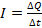(Equation 1)

The current through a resistor (with resistance R) is related to the voltage drop V across the resistor by Ohm's law: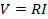(Equation 2)

Resistors in Series:

For two resistors (R1 and R2) connected in series (Figure 1), a current continuity means that the current through R1 equals the current through R2, which equals the current through both resistors. This gives: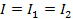(Equation 3)

Because the voltage drop across a device represents the potential difference between the two "terminals", the total voltage drop V across both resistors is the sum of the individual voltage drops across each resistor: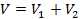(Equation 4)

Thus, with Ohm's law, the total voltage drop is equal to the effective resistance, or sum of R1 and R2, times the current: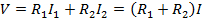(Equation 5)

Therefore, the total or "effective" resistance R of the whole series combination equals V/I. Thus, the effective resistance of resistors in series equals the sum of the individual resistances. That is,(Equation 6)

This can be also generalized to a series combination of multiple resistors. For example, if a large resistance is connected in series with a very small resistance, the total resistance will be mainly determined by the large resistor.

Further, the total current I is equal to the total voltage drop V divided by the effective resistance, or the sum of the two resistances: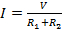(Equation 7)

Thus, the individual voltage drops (V1 and V2) can be related to the total voltage drop V as: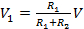(Equation 8)

and,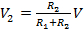(Equation 9)

This relationship describes the "voltage division," or how the voltage is divided among two series resistors proportional to the resistance.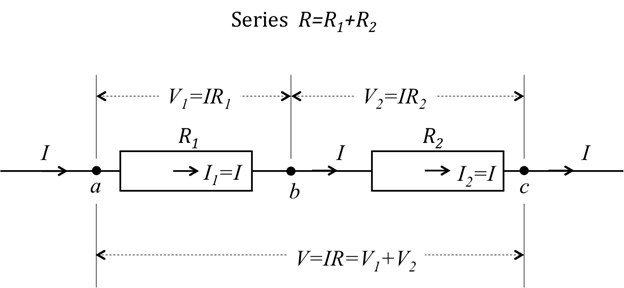Figure 1: Diagram showing two resistors connected in series.

Resistors in Parallel:

If the two resistors are instead connected in parallel, as shown in Figure 2, they share the same voltage drop V, but the total current I is split between them: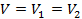(Equation 10)

and,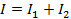(Equation 11)

Therefore:(Equation 12)

which also means that the effective resistance, R, is equal to the "product-sum" of the two parallel resistances, or: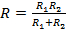(Equation 13)

Any resistor is also a conductor, and the conductance G of a resistance R is defined as the inverse of the resistance: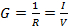(Equation 14)

where the second equality is due to Ohm's law (Equation 2).

Then for parallel resistors: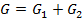(Equation 15)

If a large resistance is connected in parallel with a very small resistance, the total resistance is mainly determined by the small resistance, which has the large conductance.

For a parallel connection, the current will be divided in proportion to the conductance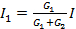, and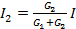(Equation 16)

which also means,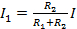, and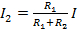(Equation 17)

(note however, the numerator is the other resistance).

For all of these examples, it is important to note that it is assumed that the wires connecting the resistors have negligibly small resistances as compared to R1 and R2. If not, the wires themselves should be modeled as resistors connected in series to R1 and R2, and add their resistances to become part of R1 and R2.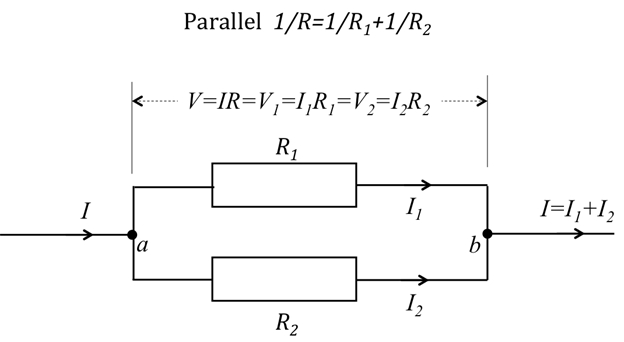Figure 2: Diagram showing two resistors connected in parallel.

Procedure

1. Practice on Generating and Measuring Current, Voltage, and Resistance

1. Obtain a current source, a voltage source, and two multimeters that can measure voltage, current, and resistance.
2. Obtain two 100-Ω resistors and two 10-Ω resistors.
3. Obtain a breadboard, which is a convenient platform to connect and organize different circuit elements. The breadboard has many groups of pins. The pins of each group are connected together at the back of the board, and wires plugged into the same group are shorted together. A wire plugged into the same group of pins as a terminal of a circuit element is connected to that terminal. Different terminals of a circuit element not meant to be shorted should be plugged into different pin groups. In the following, the action of connecting two terminals means plugging them into the same pin group (in the figures, distinctive nodes labelled "a", "b", and "c" represent distinctive pin groups on the breadboard). This is done provided that such terminals are made of wires that can be conveniently inserted into a pin hole. Otherwise, it is possible to use cables, banana plugs, and clamps to make electrical connections (for example, from a terminal on the instrument to an exposed pin leg on the breadboard).
4. Connect the output terminal of the current source to one terminal of the 100-Ω resistor, and then connect the other terminal of the resistor back to the other terminal of the current source.
5. Generate 1 mA current through the resistor.
6. Select the voltage measurement or voltmeter mode of the multimeter, and connect the two terminals of the multimeter to the two terminals of the resistor. Measure the voltage drop cross the resistor.
7. A positive reading means that the potential at the positive terminal of the multimeter is higher than that at the negative terminal. Use the voltage reading to verify the Ohm's law relationship (Figure 3).
8. Next, connect one terminal of a voltage source to one terminal of the resistor, and connect the other terminal of the resistor back to the other terminal of the voltage source. Produce a 10 V voltage across the 100-Ω resistor.
9. Use the multimeter in the current measurement or ammeter mode, and connect the probes in series with the resistor. Measure the current through the resistor.
1. A positive reading means that the electrical current is flowing from the positive terminal of the multimeter to the negative terminal. Then, verify Ohm's law (Figure 4).
2. Note that the multimeter needs to be in series with a resistor to measure its current (ammeter), but needs to be in parallel with a resistor to measure its voltage drop (voltmeter). In these measurements, assume that the "internal" resistance of the ammeter is very small but that of the voltmeter is very large. If this is not rigorously true then the current or voltage measured by the meter would be different from the value before the connection of the meter.
10. Finally, directly measure the resistance using the multimeter (Figure 5) by selecting the resistance measurement mode. Connect the two terminals of the meter with the two terminals of the resistor and read the resistance. Confirm that the resistance makes sense based on the previous current and voltage measurements.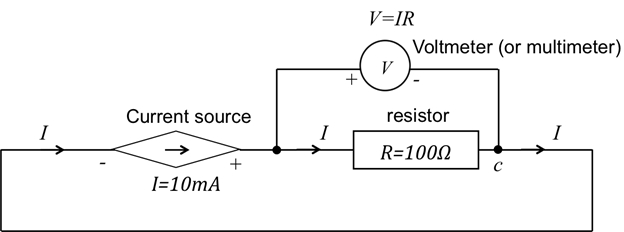Figure 3: Circuit diagram of sourcing a current through resistor R and measuring voltage.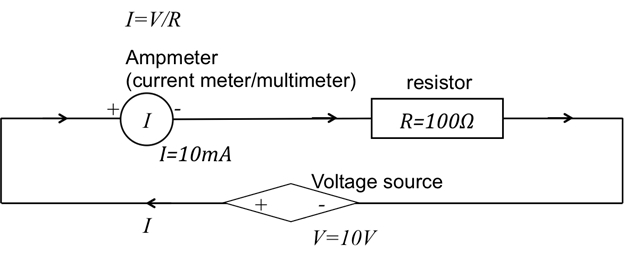Figure 4: Circuit diagram of sourcing a voltage across resistor R and measuring current.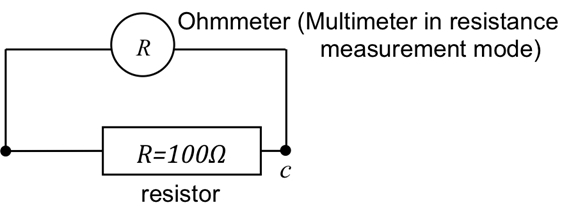Figure 5: Connection of a multimeter to measure resistance of a resistor.

2. Resistors in Series

1. Connect two 100-Ω resistors in series (refer to Figure 1). On the breadboard, this means plugging the two ends of the first resistor into two different pin groups (not shorted), say group a and b, and plugging the two ends of the second resistor into pin groups b and c (c is yet another pin group not shorted to a and b).
1. Connect a common current of 10 mA through both resistors using a current source as described in step 1.4.
2. Using a multimeter in voltmeter mode, measure the voltage drop across each resistor. Then confirm the resistance values of R1 and R2 using Ohm's law.
3. Now use the voltmeter to measure the voltage V across the entire series combination (between points a and c in Figure 1), and obtain the total series resistance.
4. Enter the measured resistance values, as shown in Table 1.
5. Disconnect the power sources and meters, then use a voltage source to provide a total of 10 V across both resistors (between points a and c).
6. Use a multimeter in ammeter mode to measure the current (I1) entering R1, and the current (I2) entering R2.
7. Verify that I1 is equal to I2 (or approximately equal; any small difference might be due to slightly different configurations, including wire resistances that changed slightly).
2. Repeat the procedure in step 2.1 for two 10-Ω resistors in series, and measure the wire resistance using the multimeter.
3. Repeat the procedure in step 2.1 for one 100-Ω resistor in series with one 10-Ω resistor, and repeat the resistance measurements.

3. Resistors in Parallel

1. Connect two 100-Ω resistors in parallel (refer to Figure 2). On the breadboard, plug the two ends of each resistor into two pin groups, say a and b.
1. Source a common voltage drop of V = 10 V using a voltage source.
2. Use a multimeter to measure the current I1 entering R1, thus confirming that R1 = V/I1. Then, measure I2 entering R2, thus confirming that R2 = V/I2.
3. Now put the amp meter in front of the parallel combination (before point a in Figure 2), and obtain the total series resistance R = V/I.
2. Repeat the above in step 3.1 for two 10-Ω resistors in parallel, and again for one 100-Ω resistor in parallel with one 10-Ω resistor.

4. LEDs in Series and Parallel Connection

1. Obtain two small LED light sources, which can be treated as two resistors.
2. Connect 1 V source to power 1 LED (similar connection as step 1.5), and observe its brightness.
3. Now connect the two LEDs in parallel between the 1 V source (similar connection as in step 3.1), and observe their brightness.
4. Now connect the two LEDs in series and between the 1 V source (similar connection as step 2.1.5), and observe their brightness again.

Simple circuits such as resistive elements connected in series or parallel are key components of complex electrical circuits.

Connecting resistors in series results in a division of voltage, while sharing the same current.

Conversely, when resistors are connected in parallel, it results in a division of current and the voltage across the resistors is the same. To understand the functioning of any electrical circuit, simple or complex, Ohm's law is used.

This video will cover the basics of connecting electrical resistive components in series and parallel, and explain the principles of calculating voltage and current for these circuits based on Ohm's law.

As per Ohm's Law, the current 'I' through a resistor of resistance 'R', is directly proportional to the voltage drop 'V' across the resistor or the voltage drop is the product of the resistance 'R' and the current 'I'.

If two resistors, R1 and R2, are connected in series, that is one after the other, then the same current flows through both resistors.

In this case, the total voltage drop 'V' is equal to the sum of the voltage drop across each resistor. On applying the Ohm's law for voltages V1 and V2, and since the currents are equal, we derive the total voltage drop as equal to the sum of resistances R1 and R2 times the current 'I'.

Moving I to the other side of the equation gives V/I, which, as per Ohm's law, is the total effective resistance of the circuit 'R'. Thus, 'R' is simply the sum of the individual resistances.

This rule is applicable for any number of resistors connected in series.

Conversely, if the two resistors, are connected in parallel, which means they are both wired between two shared terminals, then both resistors share the same voltage drop.

However, the total current 'I', is split between the resistors and is thus equal to the sum of individual currents of the circuit. Now, if we take this current equation, apply Ohm's law, cancel out the voltages on either side as they are equal for parallel resistors, and rearrange the equation for effective resistance 'R', we observe that R equals to the 'product-over-sum' of the two individual resistances

Let's go back to the equation before rearrangement. The inverse of resistance is conductance, 'G'. Therefore, for two parallel resistors, the effective conductance is equal to the sum of the two conductance values.

This rule can also be extended to multiple resistors connected in parallel.

The following experiment demonstrates the step-wise method of connecting resistors in either configuration and analyzing the circuits using the Ohm's Law.

To begin, gather the necessary materials and instruments, namely a current source, a voltage source, two multimeters, two 100-ohm resistors, two 10-ohm resistors, and a breadboard.

Using the breadboard, connect one end of a 100-ohm resistor to the output terminal of the current source. Then connect the other end of the 100-ohm resistor to the opposite terminal of the source.

Next, generate a 10-milli ampere current through the resistor and set the multimeter in voltage measurement mode. Now connect the two terminals of the multimeter to the two terminals of the resistor and measure the voltage drop across the resistor.

A positive reading means that the potential at the positive terminal of the multimeter is higher than that at the negative terminal. Using this voltage readout Ohm's law can be verified.

Next, apply a 1-volt voltage across the 100-ohm resistor. Now set the multimeter in current measurement mode. Connect the terminals of the multimeter in series with the resistor and measure the current through it.

A positive current reading means that the current is flowing from the positive terminal to the negative terminal of the multimeter. Using the measured current, Ohm's law can be verified.

Next, disconnect the voltage source from the resistor. Set the multimeter to resistance measurement mode. Connect the two terminals of the multimeter to the two terminals of the resistor and directly measure the resistance.

The measured resistance should re-verify Ohm's law and previously measured current and voltage measurements.

First, connect two 100-ohm resistors in series on the breadboard. Then connect them to the current source as described in the previous section and apply a 10-milli ampere current through both resistors.

Using the multimeter in voltmeter mode, measure the voltage drop across each resistor and across the entire series combination.

Next, apply a 10-volt voltage across both resistors. Then set the multimeter in the ammeter mode and measure the current through each resistor.

Connect two 100-ohm resistors in parallel. Then, connect them to a voltage source as described previously. Apply a 10-volt voltage across the resistors.

Using the multimeter in ammeter mode, measure the current through each resistor and through the entire parallel combination of resistors.

The brightness of lightbulbs depends not only on the voltage applied, but also on whether they are connected in series and/or with other components

Obtain two light emitting diodes or lightbulbs, each with resistance of few ohms, which can be used in place of the resistors. Connect one lightbulb to the voltage source as described previously. Apply a one-volt voltage across the lightbulb and observe its brightness.

Now, turn off the voltage source and connect the second lightbulb in parallel with the first. Apply a one-volt voltage across the parallel combination and observe the brightness of both lightbulbs.

Finally, turn off the voltage source and re-connect the lightbulbs in series. Apply a one-volt voltage across the series combination and observe the brightness of both lightbulbs.

Now that we have reviewed the protocols, let us look at the results of connecting resistors in series and parallel

The results from the series experiment show that, as per Ohm's law, the voltage across each resistor is directly proportional to the respective resistance. In addition, the voltage across both resistors is the sum of voltages across each resistor; whereas the current flowing through each resistor and through the combination is the same. Furthermore, the total resistance of the circuit is equal to the sum of the two individual resistances.

This proves the predicted voltage, current, and resistance relationships for a series combination.

On the other hand, when the resistors are connected in parallel, the current across each resistor is inversely proportional to the resistance, in accordance with the Ohm's law. Additionally, the total current flowing through the parallel combination is the sum of the individual currents, but the voltage across each resistor and across the combination is the same.

Lastly, if we convert the resistance values to conductance values, the results show that the total conductance of the circuit is equal to the sum of the two individual conductance values. This validates the theoretical voltage, current, and conductance relationships for a parallel combination.

When the lightbulbs are connected in series or parallel different levels of lightbulb brightness are observed. When lightbulbs are connected in parallel, both lightbulbs have similar brightness to the single lightbulb connected to the same voltage source.

This is because the lightbulbs are powered by voltage, and the ones connected in parallel each have the same voltage of 1 volt across them, as in the case of a single lightbulb.

On the other hand, the two lightbulbs connected in series are dimmer than the single lightbulb. This is because the two lightbulbs in series each receive only 0.5 V, as the voltage is split between them.

Series and parallel resistor connections are common in circuit applications. Let us now look at some examples where these are used.

A common application of series connection is a voltage divider circuit that converts a larger voltage into a smaller one. The input voltage is applied across two series resistors and the output voltage is obtained from the connection between them. Voltage division is the result of distributing the input voltage among the resistors of the divider circuit.

Commercial wiring in our homes, offices, labs and classrooms are setup so that multiple electrical appliances can be plugged in and used. This is possible because any electrical instrument, when plugged into the 110-volt outlet in the wall, is connected in parallel to all the other instruments that are already in use.

Thus, they all share the 110-volt common voltage and under normal operating conditions are able to operate without affecting other instruments.

You've just watched JoVE's introduction to Series and Parallel Resistors. You should now understand how to connect current and voltage sources, and measure various electrical circuit parameters using the multimeter. Additionally you should now also know how to connect resistances in series and parallel and analyze them using Ohm's Law. Thanks for watching!

Results

Representative results anticipated from the above procedure are listed below in Table 1 for resistors in series, and in Table 2 for resistors in parallel.

According to the results in Table 1, the total resistance R measured obeys Equation 6, where the resistances for components in series add to give the total resistance. The results in Table 2 show that the total resistance for resistors in parallel follows Equation 12 (or Equation 13), where the reciprocals (i.e., conductances) of the resistances in parallel add to give the total effective resistance.

When using LEDs in place of resistors, it is clear that the LEDs connected in parallel both have similar brightness to the single LED connected to the same voltage source. This is because the LEDs are powered by voltage, and the ones connected in parallel share the same voltage source (1 V in this case). Therefore, the configuration does not affect the operation of the each LED. In contrast, the two LEDs connected in series are dimmer than the single LED. This is because the two LEDs in series each receive only 0.5 V, as the voltage is split between them.

 R1 R2 Rseries 100 Ω 100 Ω 200 Ω 10 Ω 10 Ω 20 Ω 100 Ω 10 Ω 110 Ω

Table 1: Data collected for two series resistances R1 and R2 and the total effective resistance R = Rseries.

 R1 R2 Rparallel 100 Ω 100 Ω 50 Ω 10 Ω 10 Ω 5 Ω 100 Ω 10 Ω 9.1 Ω

Table 2. Data collected for two parallel resistances R1 and R2 and the total effective resistance R = Rparallel.

Applications and Summary

In this experiment, we have reviewed how to use voltage and current sources, and multimeters (voltmeter, current/amp meter, ohmmeter) to verify the current continuity law and Ohm's law. We also demonstrated how resistance adds in series connection, and how conductance adds in parallel connection.

Series and parallel connections are common in many circuit applications. For example, to use a voltage source V as a current source for some device with resistance R1, connect a much larger fixed resistance R2 with the voltage source and the device R1. Then, the current through R1 is approximately V/R2.

When any electrical appliance or equipment is plugged into a 110-V outlet on the wall, the connection is made in parallel with other instruments that may have already been plugged in. They all share the 110-V common voltage and each should operate without affecting others-within a certain range of operation conditions.

The author of the experiment acknowledges the assistance of Gary Hudson for material preparation and Chuanhsun Li for demonstrating the steps in the video.X